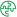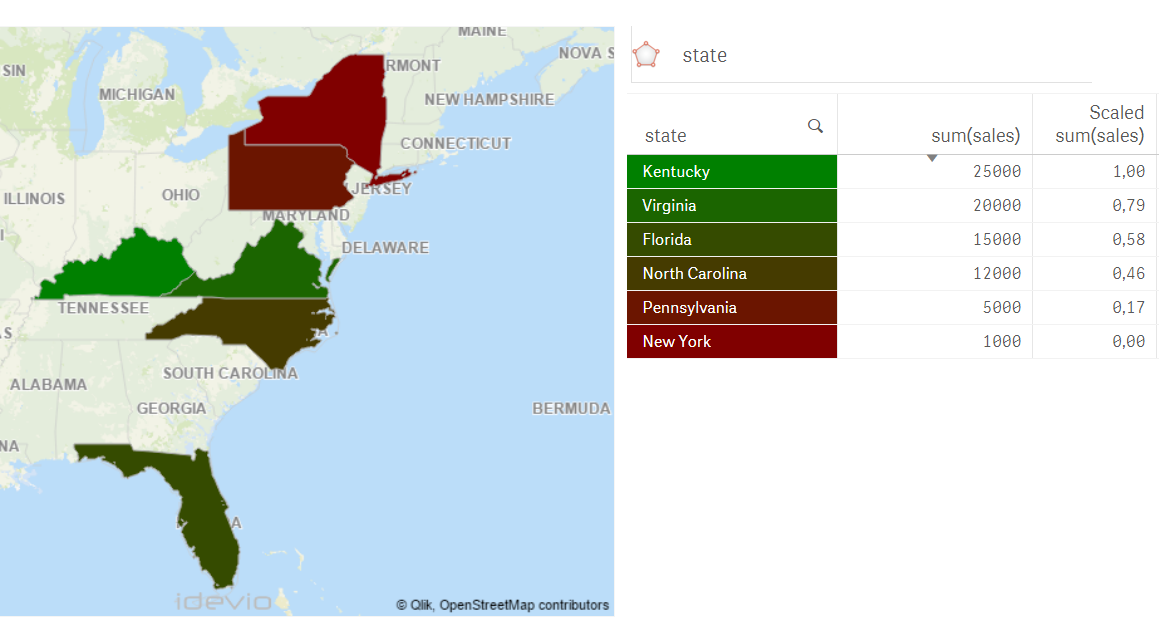# GeoAnalytics

Discussion Board for collaboration regarding Qlik GeoAnalytics.

Announcements
QlikWorld 2023, a live, in-person thrill ride. Save \$300 before February 6: REGISTER NOW!
cancel
Showing results for
Did you mean:Contributor III

## Change Color of the Area layer

Hi Guys,

We're using GEO Analytics and QlikView to plot the turnover of our customers by zipcode of the customer adress.

We would like to use a Color Scale from Red(lowest) to Green(highest). In QV the standard color modes/ shemes doesn't support this color scale. Is there an easy way to change the color scale to our requested scale?

1 Solution

Accepted SolutionsEmployee

Hello,

The idea is to convert your values to a scale between 0 and 1, the formula to do this is:

(x-min(x))/(max(x)-min(x))

x: aggr(sum(sales),zip)

min(x): min(total(aggr(sum(sales),zip)))

max(x): max(total(aggr(sum(sales),zip)))

Replacing in the formula to get the "scaled sum(sales)":

(aggr(sum(sales),zip)-min(total(aggr(sum(sales),zip))))/(max(total(aggr(sum(sales),zip)))-min(total(aggr(sum(sales),zip))))

Finally: ColorMix1((aggr(sum(sales),zip)-min(total(aggr(sum(sales),zip))))/(max(total(aggr(sum(sales),zip)))-min(total(aggr(sum(sales),zip)))),red(),green())

Here is how it looks, applied to a simple example with States:Best Regards,

Ana Yakushi

Qlik GeoAnalytics

3 RepliesEmployee

Hello Guy,

You can use this expression to define the color of the areas on the map (change the Color Mode in the Area Layer's properties to "By Expression")

=ColorMix1(value ,lowest_value_color, highest_value_color)

=ColorMix1(your_value,red(), green())

The value has to be scaled from 0 to 1.

Hope it helps.

Best Regards,

Ana Yakushi

Qlik GeoAnalyticsContributor III
Author

Hello Ana,

Thanks for your quick reply. Its almost seems to work for us. Now we're just figuring out our way to set our value correct.

There are multiple zipcodes with a sum (Sales). Like this:

Zipcode:       sum(Sales)

1                  15.000

2                  1.000

3                  25.000

Now we're looking for a way to plot zipcode '2' with color Red(Lowest sum(Sales)) and zipcode '3' with color Green(Highest sum(Sales)).

Thanks a lot!Employee

Hello,

The idea is to convert your values to a scale between 0 and 1, the formula to do this is:

(x-min(x))/(max(x)-min(x))

x: aggr(sum(sales),zip)

min(x): min(total(aggr(sum(sales),zip)))

max(x): max(total(aggr(sum(sales),zip)))

Replacing in the formula to get the "scaled sum(sales)":

(aggr(sum(sales),zip)-min(total(aggr(sum(sales),zip))))/(max(total(aggr(sum(sales),zip)))-min(total(aggr(sum(sales),zip))))

Finally: ColorMix1((aggr(sum(sales),zip)-min(total(aggr(sum(sales),zip))))/(max(total(aggr(sum(sales),zip)))-min(total(aggr(sum(sales),zip)))),red(),green())

Here is how it looks, applied to a simple example with States:Best Regards,

Ana Yakushi

Qlik GeoAnalyticsCommunity Browser